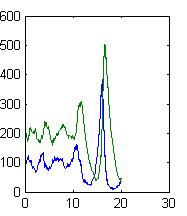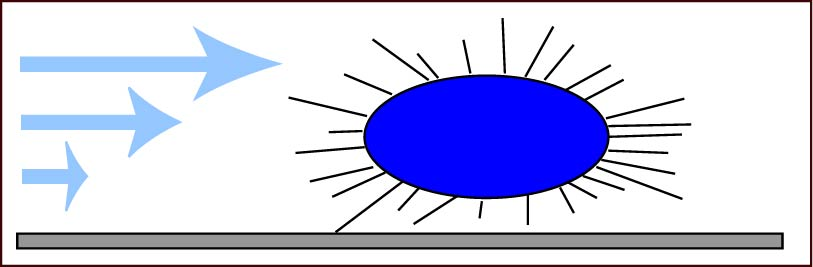# Center for Nanotechnology

## Wendy Thomas Laboratory Software

As part of an NSF CAREER Award, CMMI-0645054, the Thomas laboratory has developed stochastic simulation software as part of its research and educational efforts. This software is shared on this web site and is intended to be used by others in education and research.Stochastic simulation of the Lotka-Voltare Predator-Prey model showing the development of oscillatory behavior from a simulations that start at the equilibrium point. In contrast, the deterministic model maintains the initial conditions (not shown.)

There are many custom software packages that simulate stochastic chemical reactions, but we seek to teach students to understand the algorithms and to use them to solve problems, which requires that they understand the code. Because many students learn to program in the mathematical programming language MATLAB, we have written MATLAB code to provide a tool for learning and research.

We share here two discrete stochastic differential equation (DSDE) functions. DSDEexact uses the Exact Stochastic Algorithm (DT Gillespie, "Exact Stochastic Simulation of Coupled Chemical Reactions" Journal of Physical Chemistry 81 p. 2340, 1977) which is accurate but slow in many cases. DSDEtauleap uses the Tau Leap algorithm, merged with a discrete form of the chemical langevin equation (Gillespie: J. Chem Phys, Vol. 113, pp. 297–306, 2000) combined with a simple time-stepping algorithm, which is approximate but quite fast and robust, similar to the advantages and shortcomings of many ODE algorithms.

Because the three publications developing the methods noted above use different formalisms, we have developed a single formalism that describes chemical reactions in a way that is simple to learn and can be used by both functions. The DSDE solvers call a user-defined function defining the reaction equations, much as the ODE solvers call a user-defined function defining the differential equations. The user defines two matrices where each row defines a reaction and each column indicates a chemical. The matrix S defines the stoichiometry of substrates and P of products. A vector K then defines the rate constants of the reactions.We have simulated how bacteria adhere to surfaces in flow when they adhere through long elastic fimbriae that terminate in catch bonds, which are activated by tensile force.

We use meso-scale stochastic elastic simulations that bridge the nanoscale molecular behavior with microscale cell and even macroscale adhesive behavior. For more information, see our publication: Whitfield MJ, Ghose T, Thomas WE, "The shear-stabilized rolling behavior of E. coli examined with simulations." Biophysical Journal, 99, p. 2470-78 (2010)

Soon, we will be sharing this code, but we are still finalizing and commenting it.